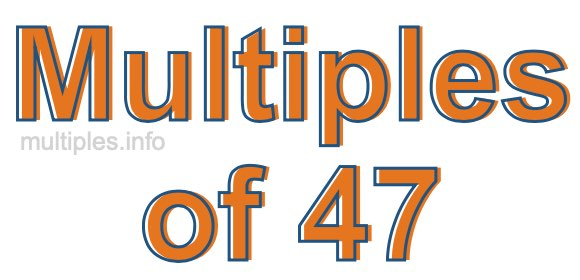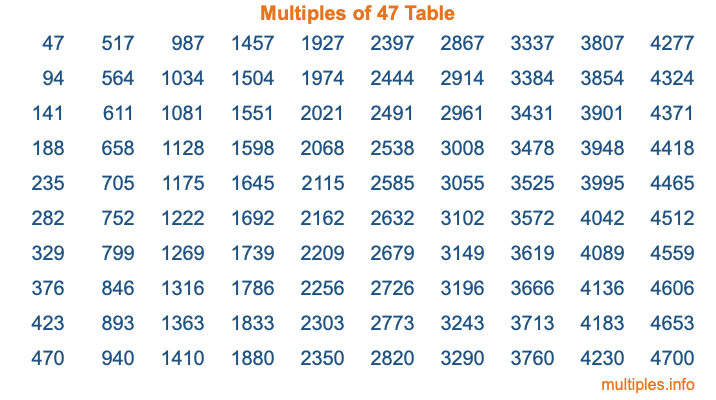Multiples of 47Welcome to the Multiples of 47 page. Here we will first teach you everything you will ever need to know about the multiples of 47, and then give you a study guide summary of everything we taught you to make sure you remember it all. Use this page to look up facts and learn information about the multiples of 47. This page will make you a multiples of forty-seven expert!

Definition of Multiples of 47
Multiples of 47 are all the numbers that when divided by 47 equal an integer. Each of the multiples of 47 are called a multiple. A multiple of 47 is created by multiplying 47 by an integer.

Therefore, to create a list of multiples of 47, you start with 1 multiplied by 47, then 2 multiplied by 47, then 3 multiplied by 47, and so on for as long as you want. Thus, the list of the first five multiples of 47 is 47, 94, 141, 188, and 235. To see a larger list of multiples of 47, see the printable image of Multiples of 47 further down on this page. We also have a category where you can choose any nth multiple of 47.

Multiples of 47 Checker
The Multiples of 47 Checker below checks to see if any number of your choice is a multiple of 47. In other words, it checks to see if there is any number (integer) that when multiplied by 47 will equal your number. To do that, we divide your number by 47. If the the quotient is an integer, then your number is a multiple of 47.

Is  a multiple of 47?

Least Common Multiple of 47 and ...
A Least Common Multiple (LCM) is the lowest multiple that two or more numbers have in common. This is also called the smallest common multiple or lowest common multiple and is useful to know when you are adding our subtracting fractions. Enter one or more numbers below (47 is already entered) to find the LCM.

Check out our LCM Calculator if you need more details about the Least Common Multiple or if you need the LCM for different numbers for adding and subtraction fractions.

nth Multiple of 47
As we stated above, 47 is the first multiple of 47, 94 is the second multiple of 47, 141 is the third multiple of 47, and so on. Enter a number below to find the nth multiple of 47.

th multiple of 47

Multiples of 47 vs Factors of 47
47 is a multiple of 47 and a factor of 47, but that is where the similarities end. All postive multiples of 47 are 47 or greater than 47. All positive factors of 47 are 47 or less than 47.

Below is the beginning list of multiples of 47 and the factors of 47 so you can compare:

Multiples of 47: 47, 94, 141, 188, 235, etc.

Factors of 47: 1, 47

As you can see, the multiples of 47 are all the numbers that you can divide by 47 to get a whole number. The factors of 47, on the other hand, are all the whole numbers that you can multiply by another whole number to get 47.

It's also interesting to note that if a number (x) is a factor of 47, then 47 will also be a multiple of that number (x).

Multiples of 47 vs Divisors of 47
The divisors of 47 are all the integers that 47 can be divided by evenly. Below is a list of the divisors of 47.

Divisors of 47: 1, 47

The interesting thing to note here is that if you take any multiple of 47 and divide it by a divisor of 47, you will see that the quotient is an integer.

Multiples of 47 Table
Below is an image of the first 100 multiples of 47 in a table. The table is in chronological order, column by column. The first column has the first ten multiples of 47, the second column has the next ten multiples of 47, and so on.The Multiples of 47 Table is also referred to as the 47 Times Table or Times Table of 47. You are welcome to print out our table for your studies.

Negative Multiples of 47
Although not often discussed or needed in math, it is worth mentioning that you can make a list of negative multiples of 47 by multiplying 47 by -1, then by -2, then by -3, and so on, to get the following list of negative multiples of 47:

-47, -94, -141, -188, -235, etc.

Multiples of 47 Summary
Below is a summary of important Multiples of 47 facts that we have discussed on this page. To retain the knowledge on this page, we recommend that you read through the summary and explain to yourself or a study partner why they hold true.

There are an infinite number of multiples of 47.

A multiple of 47 divided by 47 will equal a whole number.

47 divided by a factor of 47 equals a divisor of 47.

The nth multiple of 47 is n times 47.

The largest factor of 47 is equal to the first positive multiple of 47.

47 is a multiple of every factor of 47.

47 is a multiple of 47.

A multiple of 47 divided by a divisor of 47 equals an integer.

47 divided by a divisor of 47 equals a factor of 47.

Any integer times 47 will equal a multiple of 47.

Multiples of a Number
Here you can get the multiples of another number, all with the same attention to detail as we did for multiples of 47 on this page.

Multiples of
Multiples of 48
Did you find our page about multiples of forty-seven educational? Do you want more knowledge? Check out the multiples of the next number on our list!